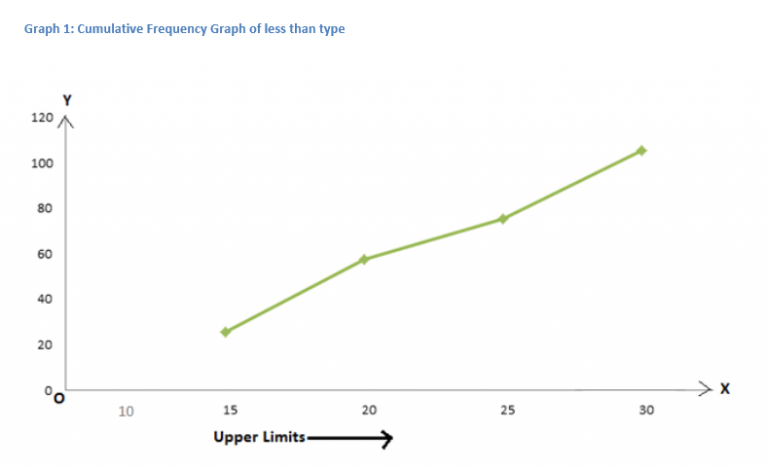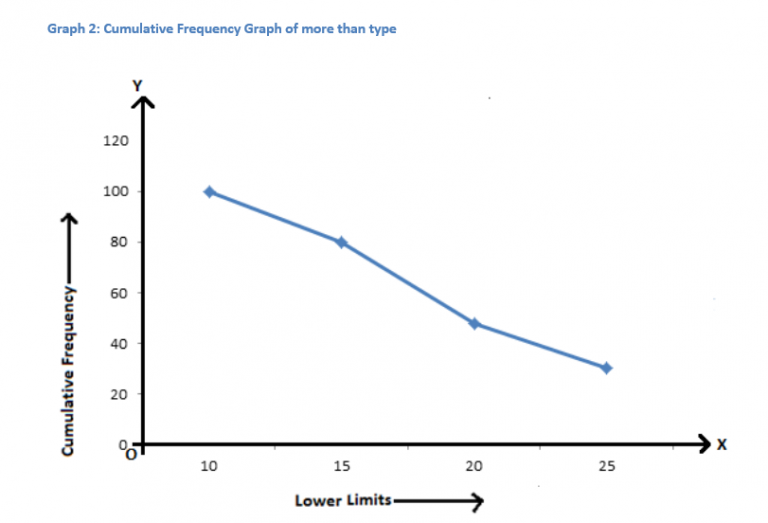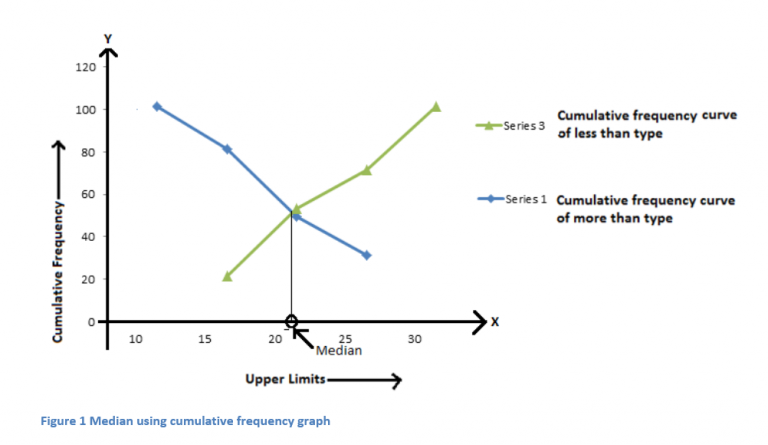# Cumulative Frequency Distribution

Representation of data in a tabular or graphical form which indicates the frequency (number of times an observation occurs within a particular interval) is known as a frequency distribution of data.

In a set of data or observations, cumulative frequency is used to determine the number of observations which lie above a specific observation. To calculate the cumulative frequency for a particular observation, frequency of that observation is added to the sum of frequencies of its predecessors. The last observation’s cumulative frequency is the sum of all the frequencies of the entire data set.

## Graphical Representation

Representation of cumulative frequency graphically is easy and convenient as compared to representing it using table, bar-graph, frequency polygon etc.

The cumulative frequency graph can be plotted in two ways:

1. Cumulative frequency distribution curve(or ogive) of less than type
2. Cumulative frequency distribution curve(or ogive) of more than type

i) To plot the ogive of less than type, a convenient scale is chosen (the scale on both axes may vary).The upper class limit is represented on the and the cumulative frequency is represented on the . The points are then plotted according to given data set and then these points are joined by free hand smooth curve. The curve so obtained gives cumulative frequency distribution graph of less than type.

To draw cumulative frequency distribution graph of less than type, consider the following cumulative frequency distribution table which gives the number of participants in any level of essay writing competition according to their age:

Table 1 Cumulative Frequency distribution table of less than type

 Level of Essay Age Group (class interval) Age group Number of participants (Frequency) Cumulative Frequency Level 1 10-15 Less than 15 20 20 Level 2 15-20 Less than 20 32 52 Level 3 20-25 Less than 25 18 70 Level 4 25-30 Less than 30 30 100

On plotting corresponding points according to table 1, we haveii) To plot the ogive of more than type, a convenient scale is chosen which may be varying on both the axes. The lower class limit is plotted on the and the cumulative frequency is represented on the . The points are then plotted according to given data set and then these points are joined by free hand smooth curve. The curve so obtained gives cumulative frequency distribution graph of more than type.

To draw cumulative frequency distribution graph of more than type, consider the same cumulative frequency distribution table, which gives the number of participants in any level of essay writing competition according to their age:

Table 1 Cumulative Frequency distribution table of more than type

 Level of Essay Age Group (class interval) Age group Number of participants (Frequency) Cumulative Frequency Level 1 10-30 More than 10 20 100 Level 2 15-30 More than 15 32 80 Level 3 20-30 More than 20 18 48 Level 4 25-30 More than 25 30 30

On plotting these points, we get a curve as shown in the graph 2.These graphs are helpful in figuring out the median of a given data set. The median can be found out by drawing both types of cumulative frequency distribution curves on the same graph. The value of of the point of intersection of both the curves gives the median of the given set of data. For the given table 1, the median can be calculated as shown: